Performance analysis of LDPC codes on OOK terahertz wireless channels
Key Laboratory of Terahertz Solid-State Technology, Shanghai Institute of Microsystem and Information Technology Chinese Academy of Science, Shanghai 200050, China

† Corresponding author. E-mail: cwang@mail.sim.ac.cn

‡ Corresponding author. E-mail: jccao@mail.sim.ac.cn

Project supported by the National Key Basic Research Program of China (Grant No. 2014CB339803), the National High Technology Research and Development Program of China (Grant No. 2011AA010205), the National Natural Science Foundation of China (Grant Nos. 61131006, 61321492, and 61204135), the Major National Development Project of Scientific Instrument and Equipment (Grant No. 2011YQ150021), the National Science and Technology Major Project (Grant No. 2011ZX02707), the International Collaboration and Innovation Program on High Mobility Materials Engineering of the Chinese Academy of Sciences, and the Shanghai Municipal Commission of Science and Technology (Grant No. 14530711300).

Abstract
Abstract

Atmospheric absorption, scattering, and scintillation are the major causes to deteriorate the transmission quality of terahertz (THz) wireless communications. An error control coding scheme based on low density parity check (LDPC) codes with soft decision decoding algorithm is proposed to improve the bit-error-rate (BER) performance of an on-off keying (OOK) modulated THz signal through atmospheric channel. The THz wave propagation characteristics and channel model in atmosphere is set up. Numerical simulations validate the great performance of LDPC codes against the atmospheric fading and demonstrate the huge potential in future ultra-high speed beyond Gbps THz communications.

1. Introduction

Along with the rapid development of terahertz (THz) sources and detectors, recent advances in THz wave technologies have attracted more and more attention due to the huge bandwidth, large capacity, and its enormous potential for applications on wireless communications. The higher carrier frequency and broader bandwidth of THz band can contain more information, and unallocated spectrum resources can avoid much interference, congestion, which are competitive advantages for ultra-high speed data transmission in comparison with microwave and millimeter communications.[1,2] However, atmospheric absorption, scattering, and scintillation will limit the THz communication transmission distance and data rate. THz beams are sensitive to the properties of the medium and will be influenced inevitably by atmospheric turbulence when propagating through the atmosphere near the ground.[3,4] The path loss and atmospheric scintillation will seriously deteriorate the signal-to-noise ratio (SNR) and degrade the bit-error-rate (BER) performance of THz wireless communication links. Scintillation theory has been proposed in many practical light propagation applications and the atmospheric turbulence is in accordance with the scintillation index. Error control coding technology can be an efficient solution to improve the system performance in similar channels.[6,7]

Low density parity check (LDPC) codes are considered to be one of the most efficient forward error correction (FEC) schemes because of their excellent performance approaching Shannon’s limit and significant low decoding complexity when decoded with the belief-propagation (BP) algorithm or sum-product algorithm (SPA). Applications of LDPC codes on optical communications have been investigated in various methods and exhibit great advantages against channel fading. However, to the best of our knowledge, there have been few papers reported about the THz channel coding technology so far. Consequently, it is obligated to apply some powerful channel coding schemes to ensure the THz wireless link quality and LDPC codes are good candidates.

In this paper, we propose an LDPC code scheme to improve the BER performance of an on-off keying (OOK) modulated THz wireless communication system based on quantum-cascade laser (QCL) as the transmitter and quantum-well photodetector (QWP) as the receiver[14,15] and verified the feasibility of the scheme. The paper is structured as follows. In Section 2, the THz wave propagation characteristics in atmosphere are analyzed and the related communication channel model is set up. Then LDPC decoder design is introduced in Section 3. In Section 4, numerical simulations of LDPC codes with soft decision decoding algorithm in different atmospherical conditions, propagation distance, and bit rate are carried out. Simulation results demonstrate the great performance of LDPC codes in future ultra-high speed THz wireless communications. Concluding remarks wrap up this paper in Section 5.

2. System and channel model

A point-to-point THz wireless communication system using intensity modulation and direct detection (IM/DD) with OOK modulation was observed and its simplified block diagram is shown in Fig. 1. The laser beams propagate along a horizontal path through a turbulence channel with additive white Gaussian noise (AWGN). The channel is assumed to be memoryless, stationary, and ergodic, with independent and identically distributed intensity fading statistics. The instantaneous received signal yk can be described by the conventional channel model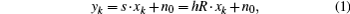where the channel state h = htha, where ht and ha are the attenuations due to the atmospheric turbulence and atmospheric absorption, respectively. xk ∈ {0, 2Pt} is the intensity of the transmitted signal with Pt being the average transmitted power of the QCL. R is the responsivity of the receiver QWP at the THz communication frequency. n0 is the signal-independent AWGN with zero mean and variance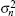. For a slow-fading channel with OOK signalling, the received electrical signal-to-noise ratio (SNR) is defined as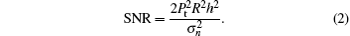Figure Option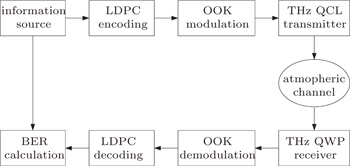Fig. 1. Atmospheric terahertz communication block scheme.

The QWP receiver noise is caused by shot noise, thermal noise and dark current. It is assumed that dark current and shot noise is negligible because thermal noise is dominated in the total noise, so the noise variancecan be expressed as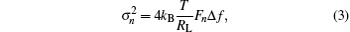where kB denotes the Boltzmann constant, T is the ambient temperature in degree Kelvin, RL denotes receiver load resistance, Fn is the amplifier noise figure and Δf is the symbol effective noise bandwidth, here marked as Δf = Rb/2, where Rb is the bit rate.

Channel characteristics at THz (0.06 THz∼1 THz) band communication has been fully studied in recent years.[17,18] In the contrary, there is no enough theory about THz wireless channel characteristics beyond 1 THz due to lack of related experimental measurements. The THz signal transmission through atmospheric channel is inevitably disrupted by atmospheric turbulence, attenuation, and geometric loss. Several statistical models have been reported to estimate the atmospheric turbulence effects, and gamma–gamma distribution has been accepted as the most suitable model for a wider range of turbulence conditions from weak to strong in various communication systems. According to the gamma–gamma model, the probability density function (PDF) of the received optical irradiance can be given by[19,20]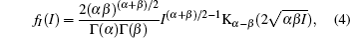where Γ(·) is the gamma function and Kv(·) is the v-order modified Bessel function of the second kind. The parameters α and β represent the effective numbers of small-scale and large-scale cells, respectively, and can be related to the atmospheric conditions. Assuming plane wave propagation and turbulent eddies of zero inner scale, these parameters can be expressed as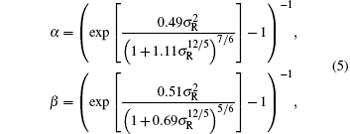where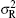is the Rytov variance and is given by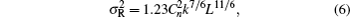where k = 2π/λ is the wave number, λ is the wave length, L is the propagation distance,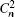is the refractive index structure constant, which is given by[3,4]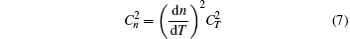with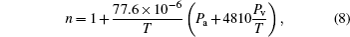where n,Pa,Pv stand for the atmospheric refraction, the atmospheric pressure, and the water vapor pressure, respectively.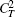is the temperature structure constant.

To simplify the discussion,is generally used as a metric of turbulence strength which is combination of propagation distance, wave length and the parameter. The evolution of the gamma-gamma models in Eq. (4) is shown in Fig. 2 when the turbulence strength varies from weak to strong with the parameter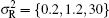.

 Figure Option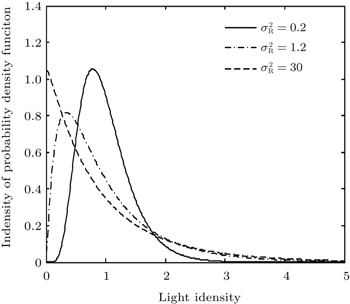Fig. 2. The probability density function (PDF) of gamma–gamma models in different turbulence conditions.

The THz communication system based on THz QCL and THz QWP uses two off-axis parabolic mirrors in the wave propagation path to collect the optical energy and change the optical path. Therefore, there is approximately no geometrical spreading loss in the system and it can be negligible. Path loss caused by atmospheric absorption and some additional loss including transmission loss through the polyethylene medium windows and reflection loss from the mirrors are considered. Then the total atmospheric attenuation can be express as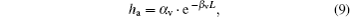where βv denotes the extinction coefficient, and αv denotes the additional loss.

3. LDPC decoder design

The LDPC code is a special linear group of codes with very sparse parity-check matrix. Many constructor methods for the parity check matrix and efficient encoding schemes with linear time have been proposed. In this paper, the quasi-cyclic LDPC codes presented by IEEE 802.16e standard are adopted to analyze the coding gain in different conditions as a reference. The LDPC codes are constructed by the base check matrix and encoded by an iterative encoding method with linear time encoding complexity.

The LDPC soft decision decoding algorithm is also a crucial point to optimize the system performance. The first step of decoding algorithm is the initialization step, which must calculate the knowledge of the log-likelihood ratio (LLR) for each received incident information bit y at the transmitted bit x = {0,1},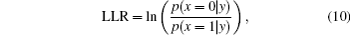where p(·) denotes the probability of the arguments. The right evaluation of Eq. (10) is the key factor of the soft decoder efficiency. It is consequently important to acquire accurate channel knowledge to correctly calculate the prior probabilities in Eq. (10).

It is assumed that perfect channel side information (CSI) denoted by h in Eq. (1) is known and is a constant in time interval of every information bit. Without loss of generality, let us assume the responsivity R = 1. In the case of OOK modulation, the atmospheric fading channel can be considered as an equivalent memoryless AWGN channel of variancewith a discrete transmitter alphabet x = {0,1} and a continuous received alphabet y, which conditional PDF of the received signal is given by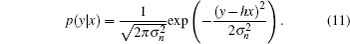Assume that “0” and “1” are transmitted in identical probability, then we can derive the priori LLR expression from Eqs. (10) and (11)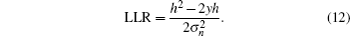After the initialization step, the iterative LDPC decoding operates by passing messages between variable nodes and check nodes according to the equivalent Tanner graph of the parity check matrix until correcting the error bits or reaching the maximum iterations.

4. Simulation and analysis

To illustrate BER performance of LDPC codes in THz wireless channel through atmosphere, plenty of numerical simulations have been carried out. The key values in the simulations are based on practical channel parameters obtained from experimental scenario, as shown in Table 1. For comparison of LDPC codes with different parameters, we considered two different code rates (R = 1/2 and R = 2/3) and two different code lengths (n = 960 and n = 1920). And different atmospheric turbulence intensities from weak to strong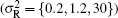are also considered. To demonstrate the improvements of performance with LDPC codes more visually, the relationship between SNR and propagation distance or data bit rate have been presented. The LLR-BP algorithm is applied to decode the LDPC codes according to the initialization formula in Eq. (12), and maximum iterations is set to 50. When the information bits are uncoded, hard decision is used for demodulation.Table 1.

Constants and system parameters.

.

The simulation results are shown in Fig. 3. Figures 3(a)3(c) correspond to weak, moderate, and strong atmospheric turbulences with parameter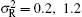, 1.2, and 30, respectively. And figure 3(d) illustrates the variation of SNR at the receiver with the propagation distance and data bit rate. From Fig. 3(a), the LDPC coded system outdistances the uncoded system on performance more than 10-dB coding gain in weak turbulence when achieving the same BER = 10−4. The coding gain of the rate 1/2 LDPC code with respect to the rate 2/3 LDPC code in the same code length 960 bits is 1.4 dB at the same BER = 10−4. When attaining BER = 10−4, the coding gain of the code length 1920 bits LDPC code is superior to that of the code length 960 bits LDPC code in the same rate 1/2 about 0.6 dB. Similar conclusions can be obtained from Figs. 3(b) and 3(c) in moderate and strong turbulences, respectively. Combined with Figs. 3(a), 3(b), and 3(c) for comparison, the BER performance of THz wireless communication system deteriorates rapidly whatever it is LDPC coded or uncoded, as the turbulence intensity gets stronger and stronger. It is obvious that the uncoded system gets worse faster than the LDPC coded system. The coding gain between 960 bits LDPC at rate 1/2 and rate 2/3 has larger difference in quite stronger turbulence conditions. In the contrary, the coding gain between 960 bits and 1920 bits LDPC at rate 1/2 exhibits no obvious changes at different atmospheric turbulence. It demonstrates that the code rate plays a more important role than the code length in excellent LDPC codes construction. From Fig. 3(d), SNR at the receiver decreases rapidly as the propagation distance or bit rate increases. In the condition of target bit rate beyond 1 Mbps, the THz wave can propagate at most 30 m due to great atmospheric absorption coefficient. When the propagation distance is over 30 m, SNR at the receiver will decrease to 10 dB or less, in which case the real signal can not be recovered from the noise. It reveals that THz wave is more suitable for short distance communications like indoor scenario. Focusing on the propagation distance at 25 m, we can see SNR at the receiver is around 20 dB and 10 dB when bit rate is 100 Mbps and 1 Gbps, respectively. In the same reason, when the bit rate reaches 10 Gbps at distance 20 m, SNR at the receiver is 15 dB. However, according to the Figs. 3(a), 3(b), and 3(c), the minimum SNR required in the THz communication systems with rate 1/2 LDPC codes reaching the best performance is 10 dB, 12 dB, and 14 dB, respectively. This means in such systems the bit rate can achieve 10 Gbps or more at communication distance 20 m. In the contrary, the minimum SNR that uncoded system requires expects to be 25 dB or more in the same distance 20 m and that directly results in the bit rate will not exceed 1 Gbps. Therefore, we can conclude that the system with LDPC codes can really increase the bit rate even beyond 10 Gbps through atmospheric wireless channel in a large degree.

 Figure Option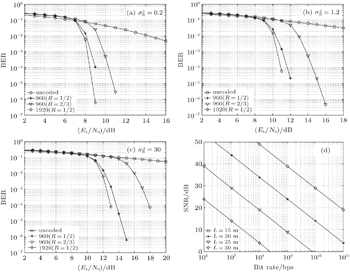Fig. 3. Performance of LDPC codes with different turbulence condition, propagation distance and bit rate. (a) Turbulence intensity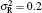, (b) turbulence intensity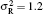, (c) turbulence intensity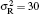, and (d) SNR of receiver with propagation distance and bit rate.

From simulation results and evaluation, we can see adverse impact caused by atmospheric absorption and scintillation is evident in THz communication system. LDPC codes can be an efficient solution to improve the BER performance and increase the communication distance and data rate. The THz wave is quite suitable for ultra-high speed indoor wireless communications and its data bit rate can be expected to exceed Gbps in LDPC coded systems. Large code length and low code rate LDPC codes are recommended in THz wireless communication. However, longer code length and lower code rate will result in a longer latency and information transfer rate. The longer block lengths increase the system implement complexity and hardware resources and reduce data throughput for high speed data transmission. Under the condition of abundant bandwidth resources, a rational LDPC code should be chosen to apply to practical THz wireless applications considering system complexity, data rate, and transmission delay, besides BER performance.

5. Conclusion

In conclusion, we present an efficient error control coding scheme based on powerful LDPC codes over a THz wireless link through atmospheric channel with THz QCL as emitter and THz QWP as detector. The introduction of LDPC codes as the channel coding is proved to be considerably significant to reduce the atmospheric attenuation and turbulence effects. Meanwhile, LDPC codes contribute great improvements on THz communication distance and data bit rate and the overall transmission rate is promised to be beyond Gbps. Though LDPC codes might have some disadvantages, they still can be a promising and potential solution to develop the practical applications in THz indoor wireless communications along with the rapid development of integrated circuit, semiconductor device, and manufacture technology.

Reference
 1 Federici JMoeller L 2010 J. Appl. Phys. 107 111101 2 Song HNagatsuma T 2011 IEEE Trans. Terahertz Sci. Technol. 1 256 3 Andrews L CPhillips R L2005Laser Beam Propagation through Random Media2nd edn.BellinghamSPIE Optical Engineering Press5874 4 Ma JMoeller LFederici J F 2015 J. Infrared Millim. Terahertz Waves 36 130 5 Rao R Z 2009 Chin. Phys. B 18 581 6 Zhu XKahn J M 2003 IEEE Trans. Commun. 51 1233 7 Xu FKhalighi ACausse PBourennane S 2009 Opt. Express 17 872 8 Gallager R G 1962 IRE Trans. Inf. Theory 8 21 9 Chung S YForney G DRichardson T JUrbanke R 2001 IEEE Commun. Lett. 5 58 10 Richardson T JUrbanke R L 2001 IEEE Trans. Inform. Theory 47 599 11 Tseng SHsieh CWang YTsai FLin K 2007 Microw. Opt. Tech-nol. 49 976 12 Sahuguede SFafchamps DAnne J VRodriguez GCances JGallion P 2009 IEEE Photon. Technol. Lett. 21 1190 13 Anguita J ADjordjevic I BNeifeld M AVasic B V 2005 J. Opt. Netw. 4 586 14 Tan Z YChen ZCao J CLiu H C 2013 Chin. Opt. Lett. 11 031403 15 Chen ZGu LTan Z YWang CCao J C 2013 Chin. Opt. Lett. 11 112001 16 Nistazakis H EKaragianni E ATsigopoulos A DFafalios M ETombras G S 2009 J. Light. Technol. 27 974 17 Chen ZCao J C 2013 Chin. Phys. B 22 059201 18 Priebe SJastrow CJacob MThomas K OSchrader TKurner T 2011 IEEE Trans. Anten. Propag. 59 1688 19 Andrews LPhilips R LHopen C Y2001Laser Beam Scintillation with Applications(SPIE Press) pp. 86–92 20 Al-Habash M AAndrews L CPhillips R L 2001 Opt. Eng. 40 1554 21 MacKay David J C 1999 IEEE Trans. Inform. Theory 45 399 22 Luby M GMitzenmacher MShokrollahi M ASpielman D A 2001 IEEE Trans. Inform. Theory 47 585 23 Richardson T JUrbanke R L 2001 IEEE Trans. Inform. Theory 47 638 24 IEEEP802.16e/D82005472480 25 Eleftheriou EMittelholzer TDholakia A 2001 Electron. Lett. 37 102